Students can download Maths Chapter 2 Real Numbers Ex 2.2 Questions and Answers, Notes, Samacheer Kalvi 9th Maths Guide Pdf helps you to revise the complete Tamilnadu State Board New Syllabus, helps students complete homework assignments and to score high marks in board exams.

## Tamilnadu Samacheer Kalvi 9th Maths Solutions Chapter 2 Real Numbers Ex 2.2

Question 1.
Express the following rational numbers into decimal and state the kind of decimal expression.
(i) $$\frac{2}{7}$$
(ii) -5$$\frac{3}{11}$$
(iii) $$\frac{22}{3}$$
(iv) $$\frac{327}{200}$$
Solution: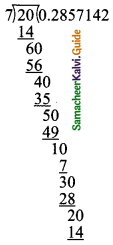(i) $$\frac{2}{7}$$ = 0.2857142….
= 0.$$\overline {285714}$$
Non-terminating and recurring decimal expansion.(ii) -5$$\frac{3}{11}$$ = -5 + 0.272 = -5.272……..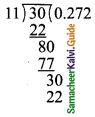= -5.$$\overline {27}$$
Non-terminating and recurring decimal expansion.

(iii) $$\frac{22}{3}$$ = 7.333……..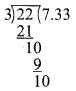= 7.$$\overline {3}$$
Non-terminating and recurring decimal expansion.

(iv) $$\frac{327}{200}$$ = $$\frac{327}{2×100}$$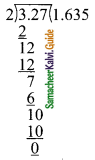= $$\frac{3.27}{2}$$
= 1.635
Terminating decimal expansion.Question 2.
Express $$\frac{1}{13}$$ in decimal form. Find the length of the period of decimals.
Solution: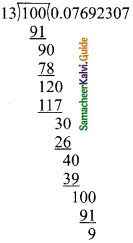$$\frac{1}{13}$$ = 0.07692307
= 0.$$\overline {076923}$$
Length of the period of decimal is 6.

Question 3.
Express the rational number $$\frac{1}{33}$$ in recurring decimal form by using the recurring decimal expansion of $$\frac{1}{11}$$. Hence write $$\frac{71}{33}$$ in recurring decimal form.
Solution: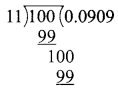$$\frac{1}{11}$$ = 0.0909……… = 0.$$\overline {09}$$
∴ $$\frac{1}{33}$$ = $$\frac{1}{3}$$ × $$\frac{1}{11}$$
= $$\frac{1}{3}$$ × 0.0909 ……..
= 0.0303 …… = 0.$$\overline {03}$$
$$\frac{71}{33}$$ = 2$$\frac{5}{33}$$ = 2 + $$\frac{5}{33}$$ = 2 + 5 × $$\frac{1}{33}$$
= 2 + 5 × 0.$$\overline {03}$$
2 + (5 × 0.030303 ……..)
2 + 0.151515 ………
2+ 0.$$\overline {15}$$
2.$$\overline {15}$$Question 4.
Express the following decimal expression into rational numbers.
(i) 0.24
Solution:
Let x = 0.242424 ………. →(1)
100 x = 24.2424 ……… →(2)
(2) – (1) ⇒ 100 x – x = 24.2424 ……….. (-)
0.2424 ……..
99 x = 24.0000
x = $$\frac{24}{99}$$
(or)
$$\frac{8}{33}$$

(ii) 2.327
Solution:
Let x = 2.327327327 ………. →(1)
1000 x = 2327.327327 ……… →(2)
(2) – (1) ⇒ 1000 x – x = 2327.327327 ……….. (-)
2.327327 ……..
999 x = 2325.000
x = $$\frac{2325}{999}$$
(or)
$$\frac{775}{333}$$(iii) – 5.132
Solution:
– 5.132 = -5 + $$\frac{1}{10}$$ + $$\frac{3}{100}$$ + $$\frac{2}{1000}$$
= $$\frac{-5000 + 100 +30 + 2}{1000}$$ = $$\frac{-4868}{1000}$$
(or)
$$\frac{-1217}{250}$$

(iv) 3.17
Solution:
Let x = 3.1777 ………. →(1)
10 x = 31.777 ……… →(2)
100 x = 317.77 …….. →(3)
(3) – (2) ⇒ 100 x – 10 x = 317.77 ……….. (-)
31.777 ……..
90 x = 286.000
x = $$\frac{286}{90}$$
(or)
$$\frac{143}{45}$$

(v) 17.215
Solution:
Let x = 17.2151515 ………. →(1)
10 x = 172.151515 ……… →(2)
100 x = 17215.1515 …….. →(3)
(3) – (2) ⇒ 1000 x – 10 x = 17215.1515 ……….. (-)
17215.1515 ……..
990 x = 17043
x = $$\frac{17043}{990}$$
(or)
$$\frac{5681}{330}$$(vi) -21.2137
Solution:
Let x = -21.213777 ………. →(1)
1000 x = -21213.777 ……… →(2)
100 x = -212137.77 …….. →(3)
(3) – (2) ⇒ 10000 x – 1000 x = -21213.777 ……….. (-)
-21213.777 ……..
9000 x = -190924
x = $$\frac{-190924}{9000}$$
(or)
$$\frac{-47731}{2250}$$

Question 5.
Without actual division, find which of the following rational numbers have terminating decimal expression.
(i) $$\frac{7}{128}$$
Solution: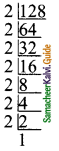$$\frac{7}{128}$$ = $$\frac{7}{2^{7}}$$
∴ $$\frac{7}{128}$$ has terminating decimal expression.(ii) $$\frac{21}{15}$$
Solution:
$$\frac{21}{15}$$ = $$\frac{7}{5}$$ = $$\frac{7}{5^1}$$
$$\frac{21}{15}$$ has terminating decimal expression.

(iii) 4$$\frac{9}{35}$$
Solution:
4$$\frac{9}{35}$$ = $$\frac{149}{35}$$
4$$\frac{149}{5×7}$$ (It is not in the form of $$\frac{P}{2^{m} × 5^{n}}$$
∴ 4$$\frac{9}{35}$$ has non-terminating recurring decimal expression.

(iv) $$\frac{219}{2200}$$
Solution: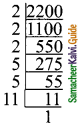$$\frac{219}{2200}$$ = $$\frac{219}{2^{3} × 5^{2} × 11}$$ (It is not in the form of $$\frac{P}{2^{m} × 5^{n}}$$
∴ $$\frac{219}{2200}$$ has non-terminating recurring decimal expression.